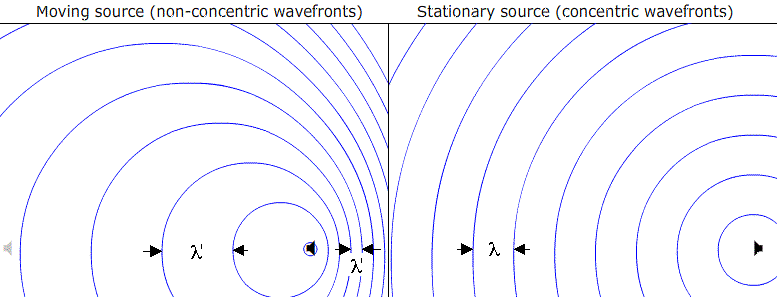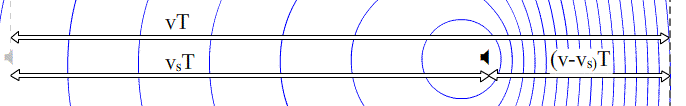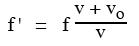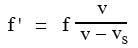## The Doppler Effect.

This page is a support page to the multimedia chapter The Doppler Effect in the volume Waves and Sound. It gives background information and further details.

#### Example of the Doppler effect

This clip (from the multimedia tutorial) shows a classic Doppler effect in sound from a moving source. A piezo-electric crystal emits a steady sound wave from the end of the handlebar of my bicyle. I approach the camera, whose microphone is fixed on a stand. A moving source and a fixed observer. The sound track gives first the sound of the stationary source, then the sound as the bicycle first approaches, then passes, then recedes from the observer. What do you hear?

One clear effect is that the sound becomes louder as the bicycle approaches and softer as it recedes. No surprise here: we've analysed this in Travelling Waves II and Radiation in three dimensions.

The Doppler effect is the change in frequency: in the case of sound, we hear this as a change in pitch: while the bike approaches the microphone, the pitch and frequency are higher than when it is stationary, when it recededs the pitch and frequency are lower.

Higher frequency means shorter period (see Oscillations to revise). On the sound track, three samples – the vertical red bars – are labelled: f for the stationary source, fv+ for approaching and fv− for receding. Later, we define velocities to be positive an object is approaching and negative if receding, hence this notation. In the bottom three graphs, the three samples have been selected and the axes expanded. (The softer distant signals have also been amplified: their amplitude has been increased, to make them easier to see.)

The arrows show 25 periods. Remembering that f = 1/T, we see that fv+  >  f   >  fv−: higher pitch on approach, lower pitch when receding. In this example, the proportional differences in frequency are only a few percent. We'll see later that this is because my speed is only a few percent of the speed of sound. However, most of us can hear this small change clearly – human hearing is very sensitive to frequency and musicians can easily notice fractions of a percent difference. Here the difference between approaching and receding is 5%, which we hear as a semitone. (You may wish to run the clip again to check.)

On a piano, all the semitones have frequency ratios of 5.9%. On most instruments, the size of a semitone can be varied according to musical context. 5% falls within this range, so we hear 5% as a semione, and some may notice that it is narrower than a piano's equal tempered semitone. (Note - frequency - note conversions here.)

#### The Doppler effect with a moving source

How does the effect shown above work? Let's imagine that the source emits a pulse of high pressure f times per second. Once the pulse leaves the speaker, it travels through the (still) air in all directions at v, the speed of sound. So, neglecting reflections, it spreads out in a sphere, as we saw in Radiation in three dimensions. In our two-dimensional diagram, we see sections of the spheres as circles. Note that each circle is centred on where the speaker was when that pulse was emitted.

Let's compare the effects of sources that are moving (left) and stationary (right), as shown below.For a stationary source (right), the circles are concentric and spaced λ apart. For the moving sour (at left), the wavefronts ahead of the source are compressed closer together. The distance between wavefronts is the wavelength so, ahead of the moving source, we observe a shorter wavelengh, λ'. Behind the moving source, the wavefronts are spread out and here the observed λ' is greater than the wavelength λ emitted by a stationary source with the same frequency.

Note the notation: we use f for the frequency emitted by the source and λ for the wavelength in still air when the source is not moving. When the source is moving, it still emits pulses at frequency f, but an observer may hear a frequency f ', corresponding to a wavelength λ' at that observer's position. The waves are travelling through air at speed v, so their frequency f ' is v/λ', where λ' is the wavelength.

In front of the source, the circles are close together, so λ' < λ. Of course, this means that the frequenrcy (remember f = v/λ) will be higher: so f ' > f ahead of the source. Behind the source, λ is increased λ' < λ and f ' > f. So the pitch is higher when the source approaches and lower when it recedes, as we heard in the example above. Let's analyse this quantitatively:

After a time T, the first circle, which is centred on the grey image of the speaker, has expanded to have a radius vT. We show this radius on the animation above. Suppose that N waves have been emitted by the time the first pulse has reached this radius. If the source is stationary (diagram at right), then the Nth wavefront is just being emitted at the position of the source, so

Nλ  =  vT  ,  so the normal wavelength   λ = vT/N .

Now look at the animation above, where we show vT and we also show the distance vsT travelled by the source moving at vs, which is less than the speed of sound v. (Note that we use subscript s for source). Between the observer and the approaching source, the waves are 'squashed together' over a distance (v − vs)T.So here we have

Nλ'  =  (v − vs)T  ,  so the wavelength ahead of the source is    λ' = (v − vs)T/N .
Dividing the equations for λ' and λ, and using f = v/λ, we have
λ'/λ  =  (v − vs)/v  ,  so    f '/f  = v/(v − vs) .
So the frequency f 'ahead of an approaching source is
f '  =  f v/(v − vs)  =  f /(1 − vs/v) .

If the source comes towards us, then vs is positive, so the frequency is increased: 1/(1 − vs/v) > 1.

What about a receding source? Here we could just make the source velocity negative, vs < 0, so the frequency would be decreased. In the diagrams above, this corresponds to an observer at left, who receives the expanded wavelengths. You may wish to go back to the first example to hear the contrast between the two, approaching then receding.

In the multimedia tutorial, we used the Doppler shift between approaching and receding to calculate the speed of the bicycle. This is an interesting experimental exercise, which we use in the laboratory section.

Before we go on, let's note that the animation is two dimensional, and could correspond to a situation in which an object moving across a pond emitted water waves. You can try this by wetting some cloth and letting drops fall into the pond as you move your hand across it. It's tricky to get regularly falling drops, however.

#### Example of the Doppler effect with moving observer

In the first example above, we had a piezo buzzer on the bicycle – a moving source – and the camera and microphone were the stationary observer. For the next clip, we've reversed the situation: the buzzer is on a microphone stand – a stationary source – and I've taped a camera with microphone onto the handlebars to give you the experience of the moving observer.

This time, in order to explain the effect, let's consider some waves we can see. Let's look at

#### The Doppler effect with water waves

Here are a couple of surfers. The one on the left is just waiting, and the red figure above him counts the waves going past. In the first part of the animation, the surfer on the right is also stationary: in the same time T, they each count the same number of waves N, so they each observe a frequency of f  =  N/T.

In the second part of the animation, however, the surfer at right starts to paddle towards the source of the waves (which must be off to the left of the animation somewhere). This means that this moving observer overtakes extra waves.

If she is paddling at speed vo (we use subscript o for observer), then in time T she travels voT. The waves have wavelength λ, so in time T she overtakes voT/λ extra waves. So the stationary observer sees a frequency f  =  N/T, while the moving observer sees a frequency

f '  =  (N + voT/λ)/T  =  N/T + vo/λ.
Now we've seen that N/T is f, the frequency with which the waves are arriving from the left. They travel at speed v and have wavelength λ, so we can write N/T  =  f  =  v/λ, which gives

f '  =  v/λ + vo/λ  =  (v/λ) (1 + vo/v)  =  f (1 + vo/v)

So the observer approaching the source of the waves sees a frequency increased by the factor (1 + vo/v)  =  (v + vo)/v.

#### More on moving observers

You might want to play the moving observer example again to hear that effect. In both that and the previous example, you'll notice that the pitch change is not abrupt: there is a smooth transition. The reason is that the stationary source is not exactly on the line of the moving observer and vice versa, for excellent safety reasons! So the component of velocity along a line joining them varies smoothly from positive to negative. The pitch glides because there is no collision.

An interesting point: At the moment of closest approach, the velocity is at right angles to the separation so, at that moment, there is no frequency shift – at least for bicycles. As we shall see later, things are sometimes more complicated when the velocities are rather large, as is often the case in cosmology.

#### Moving source and observer

We saw above that, if the observer moved with speed vo, the frequency was changed by a factor (v + vo)/v, where vo > 0 means observer approaching the source (and so an increase in frequency), i.e.We also saw that, if the source moved with speed vs, the frequency was changed by a factor v/(v − vs), where vs > 0 means source approaching the observer (and so an increase in frequency), i.e.When both source and observer are moving, both factors apply, so the general equation isNote that this expression is not symmetric in vo and vs. For small values of vo and vs, the binomial expansion of the denominator gives us an approximate expression that is symmetric:

f'  ≈  f(1 + vo/v)(1 + vs/v)  ≈  f(1 + vo/v + vs/v) ,     provided vo and vs both << v.

However, the asymmetry is clear if we consider source and observer moving at v/2: vo = v/2 gives a doppler shift of 50%, but vs = v/2 gives a shift of 100%. The asymmetry arises because we are so far considering a frame of reference in which the medium for the waves is stationary: the 'no wind case'. So let's ask:

#### What if the medium is moving?

What if there is a wind? What if the air is moving? The simplest answer is: Moving with respect to what? Yes, one can always transform to a coordinate system moving with the medium.See Relative motion.

In the animation below, the wind is moving to the right – with respect to ground, buildings and trees. However, we can use coordinates in which the air (and balloons and clouds) are stationary, and the ground etc are moving to the left. Then we can simply use the equations derived above.

Now we can think about the asymmetry again: the case with no wind, moving observer and stationary source has the same doppler shift as the stationary observer and source and wind moving with the same speed and direction.This work is licensed under a Creative Commons License.